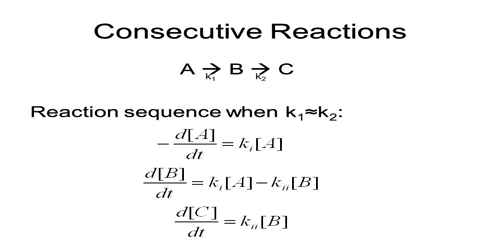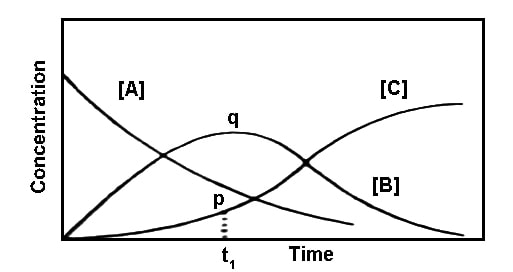# Consecutive Reactions

Consecutive reactions

Another common type of simultaneous reaction is a consecutive reaction. Many reactions which appear from a cursory glance to be simple are really consecutive reactions. In this type of reaction, an intermediate product is formed first; this intermediate product then breaks down or is converted to the final products. Such a reaction can be represented as follows:

A → k1 → B → k2 → CFig: Variation of the concentration of A, B and C with time in consecutive reaction.

The variations of the concentrations of A, B and C are schematically shown in Figure. At the start of the reaction [B] and [C] are zero. As time passes B is formed which in turn is transformed to C. Since at the beginning of the reaction the amount of B formed is quite small, the rate of formation of C is also small. This portion is shown by p in the figure. With the progress of the reaction more B is formed and the rate of the reaction increases. After the point q is reached the concentration of B falls off as indicated. Such reactions always will show a very slow rate at the beginning which is subsequently followed by more rapid rate. The time period over which the reaction is very slow is known as the induction period, as shown in Figure as t1.

The position of the maximum and its magnitude is controlled by the relative values of k1 and k2.

The overall rate of the reaction-

A → k1 → B → k2 → C

Consecutive reactions include chemical processes such as polymerization and thermal cracking and chlorination of hydrocarbons. An example of a simple consecutive reaction is the consecutive occurrence of two irreversible first-order reactions A → B → C, where A, B, and C are certain substances. The change in concentration of the substances may be calculated by integrating a system of two kinetic equations.